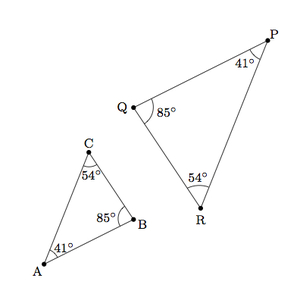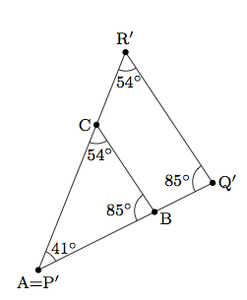# Similar Triangles I

Alignments to Content Standards: 8.G.A.5

The two triangles pictured below have three pairs of congruent angles:Are these two triangles similar?

## IM Commentary

The goal of this task is to prepare students for the angle-angle criterion for triangle similarity. Since the sum of the three angles in a triangle is always 180 degrees, having two pairs of congruent corresponding angles in two triangles tells us that the third pair of corresponding angles is also congruent. For this reason, the AAA criterion for similarity which is presented in this task is often simplified to the AA criterion for similarity. But establishing the fact that the sum of the interior angles in a triangle is 180 degrees also belongs in the eighth grade, under the same 8.G.A.5 standard. Hence it may be helpful for students to have the extra information of all three pairs of congruent angles so that they can focus entirely on the transformations that are needed to map one triangle to the other.  After working on this task, students should be ready to establish the AA criterion for triangle similarity, as presented in https://www.illustrativemathematics.org/content-standards/tasks/2042.

The fact that no side lengths are given means that students can not assign a numerical value to the scale factor relating the two similar triangles. It would be valuable for students to use dynamic software to model the transformations in the task. Without coordinates for the points, it will be difficult to determine the scale factor but students can still experiment and the software may be able to provide information about segment lengths, allowing students to calculate the scale factor explicitly.  Alternatively, this task might provide a good opportunity to have students start transitioning to more formal arguments, having them use the more precise (and abstract) value of the ratio of corresponding side lengths of the given triangles.

## Solution

Two triangles are similar when there is a sequence of translations, rotations, reflections, and/or dilations which maps one triangle to the other. These two triangles are similar. To see this, we can first move triangle $PQR$ to match up the 41 degree angles at $A$ and $P$: this can be accomplished by translating $P$ to $A$ and then using a rotation about $A$ of 180 degrees clockwise or counterclockwise. This effect of these transformations is pictured below with the image of $\triangle PQR$ denoted $\triangle P^\prime Q^\prime R^\prime$:Next we can dilation $\triangle P'Q'R'$ about $A$. If we choose a scale factor of $\frac{|AB|}{\left|P'Q'\right|}$ this will keep $A$ fixed and will map $Q'$ to $B$. We know that this dilation maps $Q'$ to $B$. Since dilation preserves angles, this dilation also map $R'$ to $C$ and $\overline{R'Q'}$ to $\overline{CB}$. Using rigid motions and a dilation we have mapped $\triangle P'Q'R'$ to $\triangle ABC$ which means they are similar.